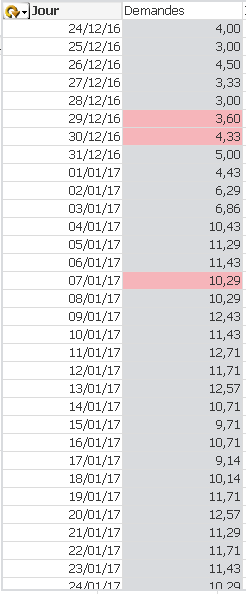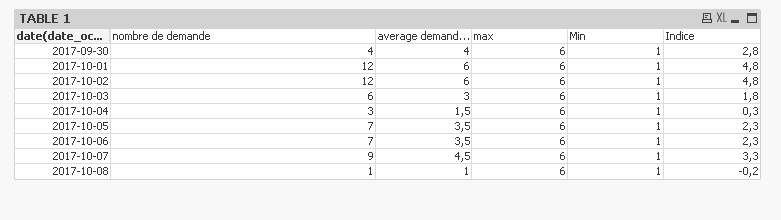# QlikView App Dev

Discussion Board for collaboration related to QlikView App Development.

Announcements
cancel
Showing results for
Did you mean:Contributor II

## Min or Max of a calculated expression

Hello,

I have been struggling too long so I ask for your kind help.

My goal is to fill in a variable with the max of the following expression. I need to get a single value at the end.

I have a table with 2 columns

dimension: it'a cyclical group with the first dimension equal to Jour=Date(date_occ-(year(date_occ)-v_AnneeRef)*364,'DD/MM/YY')

It is used to compare equivalent day between different years. A monday with a monday A tuesday with a tuesday etc

So I have several date_occ already aggregated under each "jour"

Expressions:

Demande: =(\$(vExpr_nombrededemandes))/count(DISTINCT{\$< statut_option= , statut_affaire={"*"}> } ANNEE_OCCUPATION)

vExpr_nombrededemandes= (Count({\$< libel_segment={"*"}-{'Salles expos/orgas'}-{'Autres'}, statut_option= , statut_affaire={"*"}-{'IMMOBIL'}>}  DISTINCT mot_directeur) )Thanks a lot

26 RepliesMVP

I think the problem is that you will need to use Aggr() function with the chart dimension to reach the output... but because your dimension is a calculated dimension... you cannot use it within Aggr() functionContributor II
Author

If I fix my dimension and so don't use the hierarchical group. You would have a solution?MVP

Hierarchial group is not a problem, calculated dimension behind the hierarchical group is.Contributor II
Author

Hello,

I followed your advice and I don't use anymore a calculated dimension behind the hierarchical group.

I added equivalent date thanks to an other table.You will found it in sample 2

The result I expect for the table 1 is 12 is it possible?

To put more context the goal at the end is to build an index so I will have to do

(p - pmin / (pmin-pmax)

All my value  will range between 0 and 1

thank youMVP

This gives me 12

=Max(Aggr((\$(vExpr_nombrededemandes)), [date(date_occ_equiv_2017, 'YYYY-MM-DD')]))Contributor II
Author

Thanks for the super fast answer I am gonna check that on the real model

ThanksContributor II
Author

I actually wanted to get 6 since I am looking for the max of the average per day but I did sucess on my own.

Nevertheless I still have a little issue.

The variable indeed contains the max so 6. and the min is 1, but in the "table 1" the max and the min are changing depending on the days. I want them to be fix. In order to make my "indice" between 0 and 1

Should I use a set analysis or something?MVP

Try this

=Max(TOTAL Aggr((\$(vExpr_nombrededemandes)/(count(DISTINCT{\$< statut_option= , statut_affaire={"*"}> } ANNEE_OCCUPATION))), [date(date_occ_equiv_2017, 'YYYY-MM-DD')]))

=Min(TOTAL Aggr((\$(vExpr_nombrededemandes)/(count(DISTINCT{\$< statut_option= , statut_affaire={"*"}> } ANNEE_OCCUPATION))), [date(date_occ_equiv_2017, 'YYYY-MM-DD')]))Contributor II
Author

We are really close,

Does TOTAL exclude all the set analysis I put in m formula?

In my real model value are changing when I add some filters they shoudlnt.MVP

TOTAL doesn't exclude set analysis.... can you share by an image as to what happens with set analysis add in?